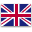UFO Battery is a professional supplier of lithium-ion batteries and power solutions.English
Home > RESOURCES > Knowledge Base > How do you size a solar panel battery?
Bll Categories
More
Latest Blog
More
Blog Tag
More

# How do you size a solar panel battery?

by：hqt

Before sizing a lithium battery for solar system, it is better to figure out the amp-hour needs first. It is a decisive factor to choose the right size of the inverter and capacity of the lithium solar battery. After calculating the daily energy use, you also need to decide the energy goal that how many days you need the battery to last as the backup power. Let’s check how to do the calculation together.

Inverter size

The inverter size is decided by the peak load of a household, and you can calculate by adding up all the wattage of various appliances that run altogether. That is to say, the inverter size must be bigger than the total wattage of all the appliances. For example, if the PC runs for 4 hours a day and the wattage is 400 watts, and you have three lights rated at 50 watts to be on for five hours, then the total daily energy use will be 400+50X3=550 watts. As a result, the inverter size is at least 550 watts.

Daily energy use

To sum up the daily energy use, you need to calculate how long each appliance or device run in a day, and multiple the wattage by the time. Considering the efficiency loses, the real value will be the calculation result multiplying by 1.5. Take the same example above, the ideal daily energy use is 400X4+50X3X5=2,350 watt-hours, and the real daily energy use is 2,350X1.5=3,525 watt-hours.Energy goal

Considering the size of your home appliances and how long you want the backup energy to last, it can be several hours to a few days. Suppose the goal is two days, and the appliances are the computer and three lights.

Battery capacity

As for the calculation of power lithium battery, it is supposed to calculate lithium battery for inverter especially when the voltage is decided by the output of the solar panels in your home solar system.

Take the daily energy use and multiply it by the energy goal which is two days, and this can be 50% depth of discharge on your lithium battery for solar system, so as to maintain the battery for longer cycle life. The battery energy should be 3.525kWhX2X2=14.1kWh, and it is divided by the voltage of the solar system so as to convert it to amp hours. If you choose 48V, then the minimum AH capacity is 14100/48=293.75Ah. To figure out how many batteries you need and the size for each one, you can divide the Ah result by the battery rating.

Chat Online 编辑模式下无法使用
Chat Online inputting...